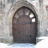BookmarkSubscribe
Choose Language Hide Translation Bar

How to get a p-value on descriptive statistics? How to get Cramer's V statistic?

Hi,

I'm new to JMP and I'm using for my master thesis. I have previously used Statview which was a much smaller programe and easier to use. I will start to do the easy part with constructing the descriptive statistics, like how many articles were published on different weekdays during the five years I have studied. It's pretty easy to get the values and percentage, but the p-value? I know that my teacher will demand me to have that in the thesis. There is something called StdErr Prob, is that the same thing as P-value? And if so it seems to calculate it only for each weekday and not the total p-value because that is only given as 0,000000000.

And in my last thesis I used something called Cramer's V, that doesn't seem to exist but it is maybe called something else in JMP?

I hope some helpful soul could give some tips

yours

ed

1 ACCEPTED SOLUTION

Accepted Solutions
Highlightedsusan_walsh1
Staff (Retired)

Re: Beginners question, how to get p-value on descriptive statistics?

The StdErr Prob is not the same as the p-value. p-values are generally labeled as Prob > Chisq or Prob > t. How to find the p-value for your analysis will depend on the platform you are using. You can find information in the JMP documentation, in this case most likely the Basic Analysis book, which can be found by selecting Help -> Books -> Basic Analysis. You can also find the documentation on the JMP website: https://www.jmp.com/en_us/support/online-help.html.

Cramer's V statistic is not given automatically in JMP. However, JMP does provide the necessary statistics in the Contingency platform to compute it by hand.

For 2x2 tables, V = (n11*n22 - n12*n21)/sqrt(n1.*n2.*n.1*n.2) , where n.i are the column totals and ni. are the row totals.
For other size tables, V = sqrt [(Qp/n)/min(R-1,C-1)] (where Qp=Pearson Chi-square statistic, R is the number of rows, and C is the number of columns).

3 REPLIES 3pmroz
Super User

Re: Beginners question, how to get p-value on descriptive statistics?

When all else fails rtfm

Launch JMP
Click on Help > Books > JMP Stat and Graph Guide
In the PDF file hit CTRL-F and type p-value

Re: Beginners question, how to get p-value on descriptive statistics?

Thanks PMroz, i solved the p-value problem.

But i still can't find Cramer's V.

http://changingminds.org/explanations/research/analysis/cramers_v.htm

SPPS has Cramer's V.

Can it have another name in JMP?

What do you guys do when you need Cramer's V, maybe other software?

Please, hope someone can help me.

ed
Highlightedsusan_walsh1
Staff (Retired)

Re: Beginners question, how to get p-value on descriptive statistics?

The StdErr Prob is not the same as the p-value. p-values are generally labeled as Prob > Chisq or Prob > t. How to find the p-value for your analysis will depend on the platform you are using. You can find information in the JMP documentation, in this case most likely the Basic Analysis book, which can be found by selecting Help -> Books -> Basic Analysis. You can also find the documentation on the JMP website: https://www.jmp.com/en_us/support/online-help.html.

Cramer's V statistic is not given automatically in JMP. However, JMP does provide the necessary statistics in the Contingency platform to compute it by hand.

For 2x2 tables, V = (n11*n22 - n12*n21)/sqrt(n1.*n2.*n.1*n.2) , where n.i are the column totals and ni. are the row totals.
For other size tables, V = sqrt [(Qp/n)/min(R-1,C-1)] (where Qp=Pearson Chi-square statistic, R is the number of rows, and C is the number of columns).